# Non-Parametric Bayesian - Subsystem Tests

This example appears in the Life Data Analysis Reference book.

You can use the non-parametric Bayesian method to design a test for a system using information from tests on its subsystems. For example, suppose a system of interest is composed of three subsystems A, B and C -- with prior information from tests of these subsystems given in the table below.

Subsystem Number of Units (n) Number of Failures (r)
A 20 0
B 30 1
C 100 4

This data can be used to calculate the expected value and variance of the reliability for each subsystem.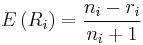$E\left(R_{i}\right)=\frac{n_{i}-r_{i}}{n_{i}+1} \,\!$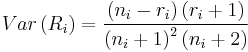$Var\left(R_{i}\right)=\frac{\left(n_{i}-r_{i}\right)\left(r_{i}+1\right)}{\left(n_{i}+1\right)^{2}\left(n_{i}+2\right)} \,\!$

The results of these calculations are given in the table below.

Subsystem Mean of Reliability Variance of Reliability
A 0.952380952 0.002061
B 0.935483871 0.001886
C 0.95049505 0.000461

These values can then be used to find the prior system reliability and its variance: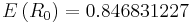$E\left(R_{0}\right)=0.846831227\,\!$$\text{Var}\left(R_{0}\right)=0.003546663\,\!$

From the above two values, the parameters of the prior distribution of the system reliability can be calculated by: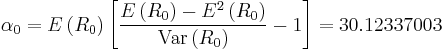$\alpha_{0}=E\left(R_{0}\right)\left[\frac{E\left(R_{0}\right)-E^{2}\left(R_{0}\right)}{\text{Var}\left(R_{0}\right)}-1\right]=30.12337003\,\!$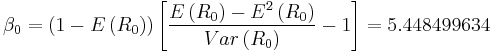$\beta_{0}=\left(1-E \left(R_{0}\right)\right)\left[\frac{E\left(R_{0}\right)-E^{2}\left(R_{0}\right)}{Var \left(R_{0}\right)}-1\right]=5.448499634\,\!$

With this prior distribution, we now can design a system reliability demonstration test by calculating system reliability R, confidence level CL, number of units n or number of failures r, as needed.

Solve for Sample Size n

Given the above subsystem test information, in order to demonstrate the system reliability of 0.9 at a confidence level of 0.8, how many samples are needed in the test? Assume the allowed number of failures is 1.

Using Weibull++, the results are given in the figure below. The result shows that at least 49 test units are needed.